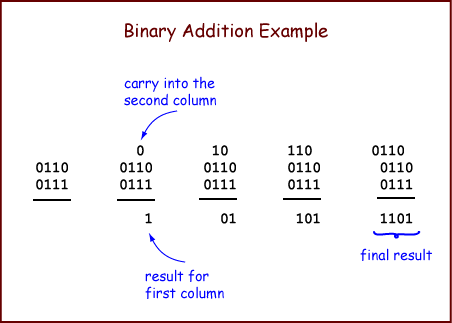```  1             0             1
0             1             1
1             0             0
10            01            10
```

Adding a column of bits is as easy as counting. It is also easy to do electronically. When you take a course in digital logic you will probably build a circuit to do it.

Now let us look at the full, n-bit, binary addition algorithm. The algorithm takes two operands and produces one result. An operand is the data that an algorithm operates on.

To add two N-bit (representations of) integers: Proceed from right-to-left, column-by-column, until you reach the left-most column. For each column, perform 1-bit addition. Write the carry-out of each column above the column to its left. The bit is the left column's carry-in.The example adds two 4-bit operands. The initial conditions are shown on the left. First, add up the bits in the right-most column. There is no carry-in to this column, so all you need to do is count the bits. The result is 1 with a carry of 0. Write the 1 as the result for the column, and put the carry above the next column. The picture shows this in blue. Continue right-to-left through the columns until all columns have been added. The carry-out of the right-most column should not go into the sum, but should be written to the right of the other carry bits.

### QUESTION 3:

Confirm that this addition is correct. (1) Check that the binary addition algorithm was performed correctly by checking the left column, then (2) translate the binary operands into decimal to fill the blanks in the right column, and then (3) verify that the decimal addition in the right column gives the same sum.

``` 0110     =      (base 10)

0111     =      (base 10)
______

1101     =      (base 10)
```

Hopefully, the binary result in the bottom row will represent the same integer as the decimal result in the bottom row.